# matheagle

•### Vertical graph transformations

Activity

matheagle

•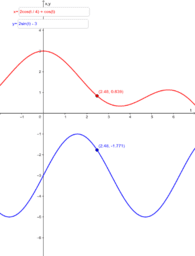### Parametrization side-by-side

Activity

matheagle

•### Linear transformation (with image of a vector u)

Activity

matheagle

•### Phase portrait: Example 3

Activity

matheagle

•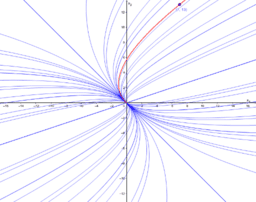### Phase portrait: Example 2

Activity

matheagle

•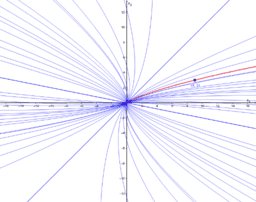### Phase portrait: Example 1

Activity

matheagle

•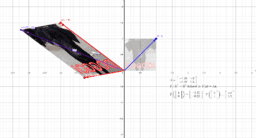### Eigenvector of a matrix used as standard matrix of a linear transformation

Activity

matheagle

•### Eigenvector of a matrix used as standard matrix of a linear transformation

Activity

matheagle

•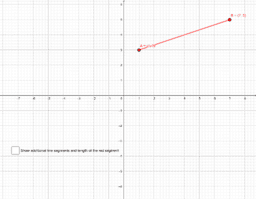### Distance formula: Length of line segment

Activity

matheagle

•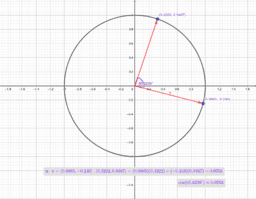### Angle and dot product (unit vectors)

Activity

matheagle

•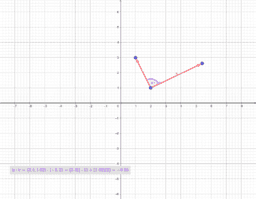### Angle and dot product (not at origin)

Activity

matheagle

•### Taylor polynomial

Activity

matheagle

•### Plane

Activity

matheagle

•### Scalar multiplication

Activity

matheagle

•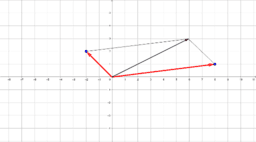Activity

matheagle

•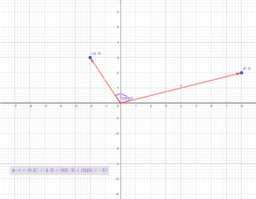### Angle and dot product

Activity

matheagle

•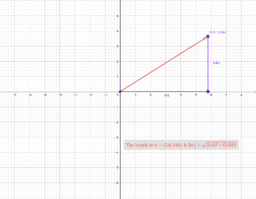### Length of vector

Activity

matheagle

•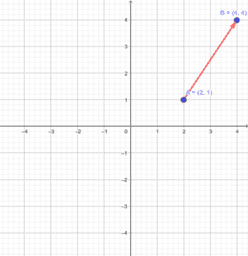### Vector determined by two points

Activity

matheagle

•### Linear transformation

Activity

matheagle

•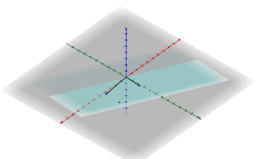### Span of two vectors in \$R^3\$

Activity

matheagle

•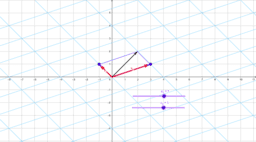### Linear combination

Activity

matheagle

•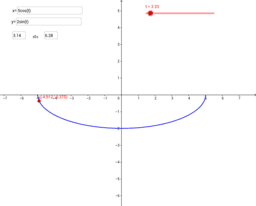### Parametrization

Activity

matheagle

•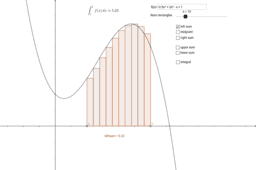### Riemann Sums and Definite Integral

Activity

matheagle

•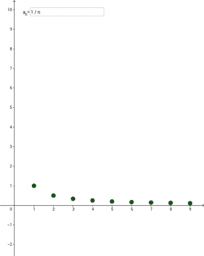Activity

matheagle